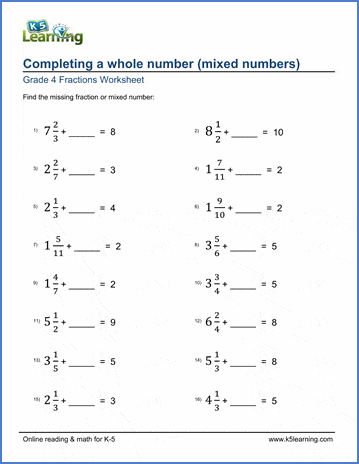# Completing a whole number

## Worksheets: Completing a whole number (adding mixed numbers)

Below are six versions of our grade 4 fractions worksheet on adding mixed numbers to complete a whole number. Students must find the fraction or mixed number missing from the addition equation. All sums are whole numbers between 1 and 10.  Answers are given as mixed numbers without simplification.  These worksheets are pdf files.## More fractions worksheets

Explore all of our fractions worksheets, from dividing shapes into "equal parts" to multiplying and dividing improper fractions and mixed numbers.

## What is K5?

K5 Learning offers reading and math worksheets, workbooks and an online reading and math program for kids in kindergarten to grade 5.  We help your children build good study habits and excel in school.